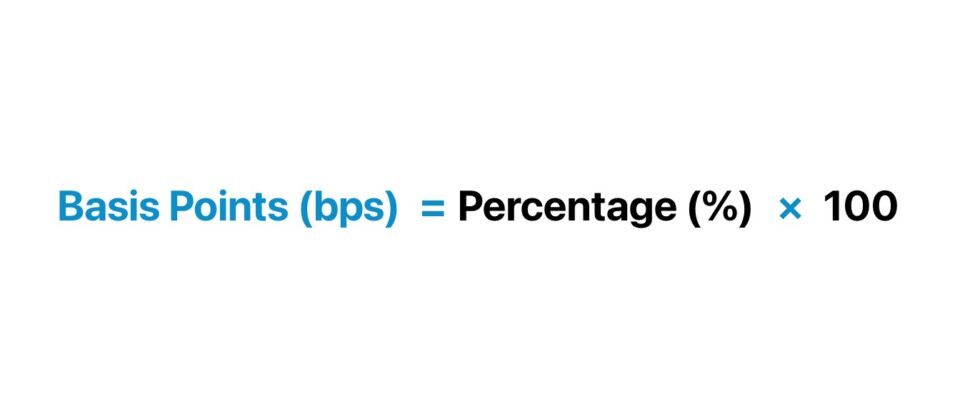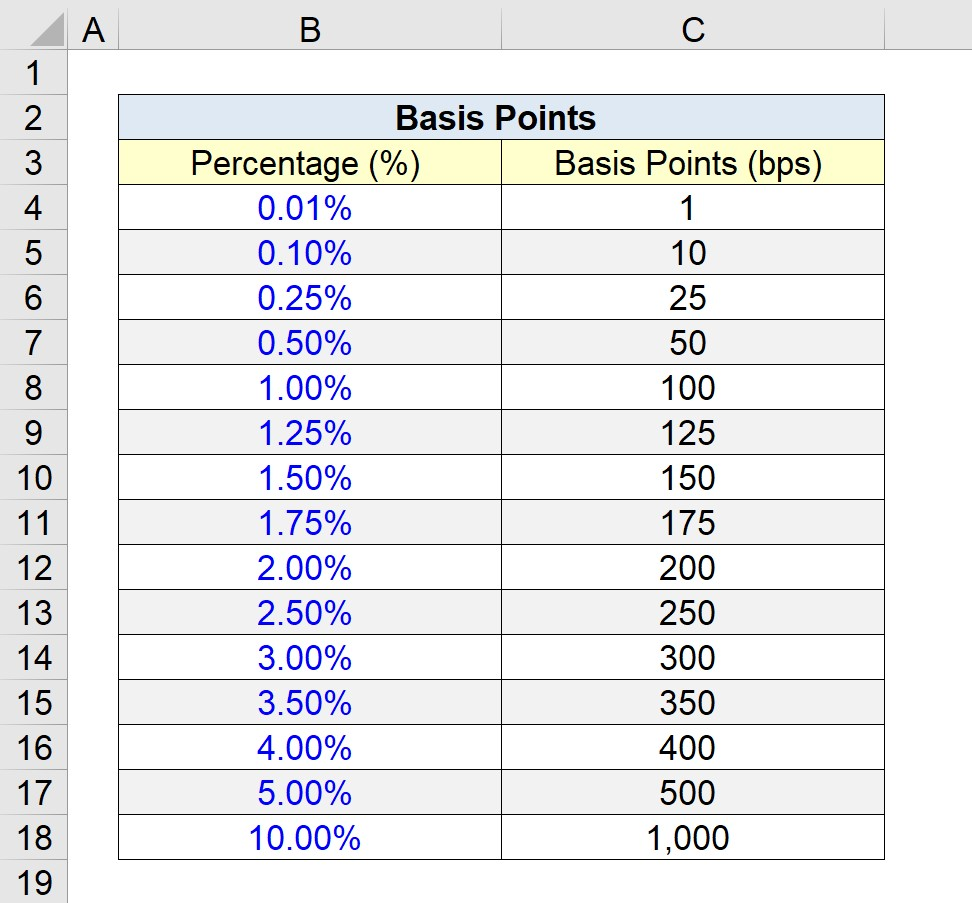Welcome to Wall Street Prep! Use code at checkout for 15% off.# Basis Points (bps)

Guide to Understanding Basis Points (bps)## How to Calculate Basis Points?

One basis point equals one-hundredth of a percentage point, or expressed numerically, 1/100th of 1.0%.

Within the finance industry, it is the norm to discuss interest rates in terms of basis points rather than percentages, especially regarding smaller figures. Using bps can be more convenient and reduce the chance of misinterpretations, as the expression is an absolute figure and is thus easier to understand than a small percentage.

Describing interest rates, spreads, and yields in terms of basis points tends to be more precise, as the implications of such minor changes can often be significant on the economy or instrument in question.

Basis points, or “bps”, are pronounced as “bips” and are relevant when speaking about a wide variety of financial instruments such as government bonds (e.g. treasury bonds, treasury bills), corporate bonds, and common shares.

While 1/100th of 1.0% might initially sound like a minuscule difference, the economic implications and impact on yields can be substantial.

For example, interest rate adjustments by the central government (i.e. the Fed in the U.S.), even by one mere basis point, could have a substantial wave of effects on the bond and equities market, contrary to what some might anticipate.

Hence, using bps ensures clarity in speech.

## How to Convert Basis Points to Percentage

In practice, the bps metric is frequently used to signify the percentage changes or yield spreads in financial instruments such as fixed income, particularly in instances wherein the difference in the interest rate is sub-one percent (<1.0%).

Basis Points Conversion Table
Percentage (%) Basis Points (bps)
0.01% 1
0.10% 10
0.25% 25
0.50% 50
1.00% 100
1.25% 125
1.50% 150
1.75% 175
2.00% 200
2.50% 250
3.00% 300
3.50% 350
4.00% 400
5.00% 500
10.00% 1,000

## Basis Points Formula (bps)

To reiterate from the earlier section, 100 bps equals 1.0%. Therefore, in order to convert the number of bps to a percentage figure, the bps must be divided by 100, as shown in the equation below.

Percentage (%) = Basis Points (bps) ÷ 100

In contrast, converting a percentage into bps — the far more common calculation — involves multiplying the percentage rate by 100.

Basis Points (bps) = Percentage (%) × 100

By expressing the percentage in the form of basis points, the incremental changes, such as the spread on bond yields, are easier to discuss, and the probability of misinterpretation is reduced.

So in order to move from bps to percentages, we divide by 100, and to switch from percentages to bps, we must multiply by 100.

## Basis Points Calculator (bps)

We’ll now move to a modeling exercise, which you can access by filling out the form below.Submitting...

## 1. Basis Point Calculation Example

Suppose we are tasked with manually building a table that converts percentages (%) to basis points (bps), similar to the above.

As we went over earlier, in order to move from percentage form to bps, we multiply the percentage on the left column by 100%, i.e. 10,000.

For instance, 0.01% converts to 1 basis points (bps).

• 0.01% × 10,000 = 1 bps

We’re moving the decimal in the percentage to the right by two places, but we must be careful not to multiply by 100% or 1, as the resulting amount will be equal to the percentage.

Conversely, we could also divide the left column by 0.01% to arrive at the same figures.

While less intuitive on paper, the latter method is arguably more convenient in Excel because there is less chance of an error related to the sign convention.

## 2. BPS to Percentage Conversion Table

We’ll wrap up our quick exercise by confirming that 100 bps equals 1.0%.

• 100 bps ÷ 10,000 = 1.0%

In closing, a screenshot of the completed spreadsheet can be found below, where we converted the percentages (%) to basis points (bps), and vice versa.Step-by-Step Online Course

### Everything You Need To Master Financial Modeling

Enroll in The Premium Package: Learn Financial Statement Modeling, DCF, M&A, LBO and Comps. The same training program used at top investment banks.